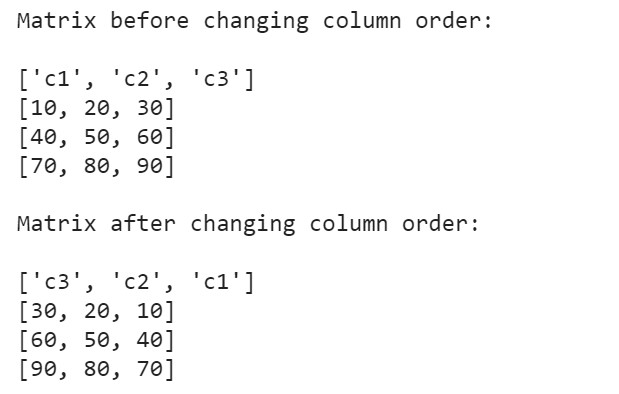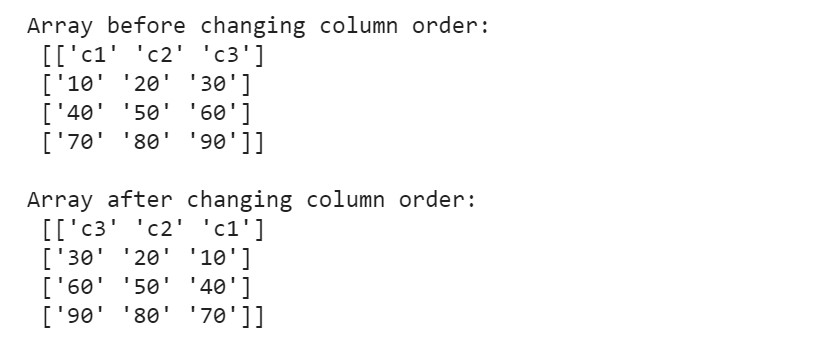# How to reverse column order in a matrix with Python?

• Last Updated : 08 Sep, 2021

In this article, we will see how to reverse the column order of a matrix in Python.

Examples:

```Input:
arr = [[10,20,30],
[40,50,60],
[70,80,90]]
Output:
30 20 10
60 50 40
90 80 70

Input:
arr = [[15,30],
[45,60],
[75,90],
[105,120]]
Output:
30 15
60 45
90 75
120 105```

Matrices are created in python by using nested lists/arrays. However, a more efficient way to handle arrays in python is the NumPy library. To create arrays using NumPy use this or matrix in python once go through this.

Method 1:

1. Iterate through each row
2. For every row, use list comprehension to reverse the row (i.e. a[::-1])
3. Append the reversed rows into a new matrix
4. Print the matrix

Example:

## Python3

 `# creating a 3X4 matrix using nested lists``matrix_1 ``=` `[[``'c1'``, ``'c2'``, ``'c3'``],``            ``[``10``, ``20``, ``30``],``            ``[``40``, ``50``, ``60``],``            ``[``70``, ``80``, ``90``]]` `# creating an empty array to store the reversed column matrix``matrix_2 ``=` `[]` `# looping through matrix_1 and appending matrix_2``for` `i ``in` `range``(``len``(matrix_1)):``    ``matrix_2.append(matrix_1[i][::``-``1``])` `print``(``'Matrix before changing column order:\n'``)``for` `rows ``in` `matrix_1:``    ``print``(rows)``print``(``'\nMatrix after changing column order:\n'``)``for` `rows ``in` `matrix_2:``    ``print``(rows)`

Output:Method 2:

An array object in NumPy is called ndarray, which is created using the array() function. To reverse column order in a matrix, we make use of the numpy.fliplr() method. The method flips the entries in each row in the left/right direction. Column data is preserved but appears in a different order than before.

Syntax:  numpy.fliplr(m)

Parameters: m (array_like) – Input array must be at least 2-D.

Returned Value: ndarray – A view of m is returned with the columns reversed, and this operation’s time complexity is O(1).

Example:

## Python3

 `import` `numpy as np` `# creating a numpy array(matrix) with 3-columns and 4-rows``arr ``=` `np.array([``    ``[``'c1'``, ``'c2'``, ``'c3'``],``    ``[``10``, ``20``, ``30``],``    ``[``40``, ``50``, ``60``],``    ``[``70``, ``80``, ``90``]])` `# reversing column order in matrix``flipped_arr ``=` `np.fliplr(arr)` `print``(``'Array before changing column order:\n'``, arr)``print``(``'\nArray after changing column order:\n'``, flipped_arr)`

Output:Flipped_arr contains a reversed column order matrix where the column order has changed from c1,c2,c3 to c3,c2,c1, and the elements of each column remain intact under their respective headers (c1,c2,c3).

My Personal Notes arrow_drop_up